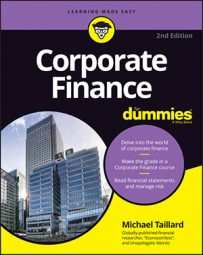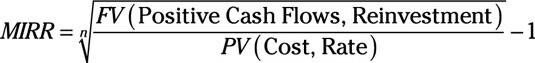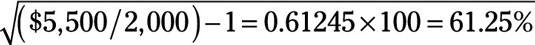##### Corporate Finance For DummiesThe accounting rate of return is helpful, but it’s so simple that it’s extremely limited in its ability to provide you with information that’s useful in your attempt to manage assets, investments, and projects. For that, you have something called the modified internal rate of return (MIRR). Modified!? Yeah.

The internal rate of return (IRR) is a good equation, but it has some faults that are easily rectified, so no one really even brings it up anymore.

The IRR is a calculation that attempts to take the net future cash flows of a project (all the positive and negative cash flows of the project) and the discount rate at which the present value of the net cash flows is zero.

Think of it like this: A project is worth \$0 at the beginning because it hasn’t produced anything. So in order to determine the IRR, you attempt to calculate the rate at which the net present value of future cash flows is 0. That rate is the IRR.

There are a couple problems with the IRR, though:

• It automatically assumes that all cash flows from the project are reinvested at the IRR rate, which isn’t realistic in most cases.

• It has difficulty comparing projects that have differing durations and cash flows.

Otherwise, the IRR can be used to evaluate a single project or single cash flow.

The MIRR tends to be more accurate in its costs and profitability of projects, though, and because the MIRR is a more robust equation with wider applications. You use the following equation to calculate the MIRR:where:

• n = number of periods

• FV = Future value

• PV = Present value

• Positive Cash Flows = The revenues/value contributions to revenues from the project

• Reinvestment = The rate generated from reinvesting future cash flows

• Cost = The investment cost

• Rate = The rate of financing the investment

• 1 = A number

Most of the time, the reinvestment rate of MIRR is set at the corporation’s cost of capital. Of course, that depends a lot on how efficient the corporation is in its financial management, so keep it an open variable based more on evaluations of the corporations’ financial performance.

Anyway, the following quick example shows you how to calculate the MIRR of a project.

Say that a project lasting only two years with an initial investment cost of \$2,000 and a cost of capital of 10 percent will return \$2,000 in year 1, and \$3,000 in year 2.

Reinvested at a 10 percent rate of return, you compute the future value of the positive cash flows as follows:

\$2,000(1.10) + \$3,000 = \$5,200 at the end of the project’s lifespan of two years.

Now you divide the future value of the cash flows by the present value of the initial cost, which is \$2,000, to find the geometric return for two periodsNote that this calculation doesn’t take a financing cost into account. That’s okay, because most corporations can afford \$2,000 with no problem. Also note that had you used the IRR instead of the MIRR, the rate of return would have been substantially higher, but also substantially less accurate.